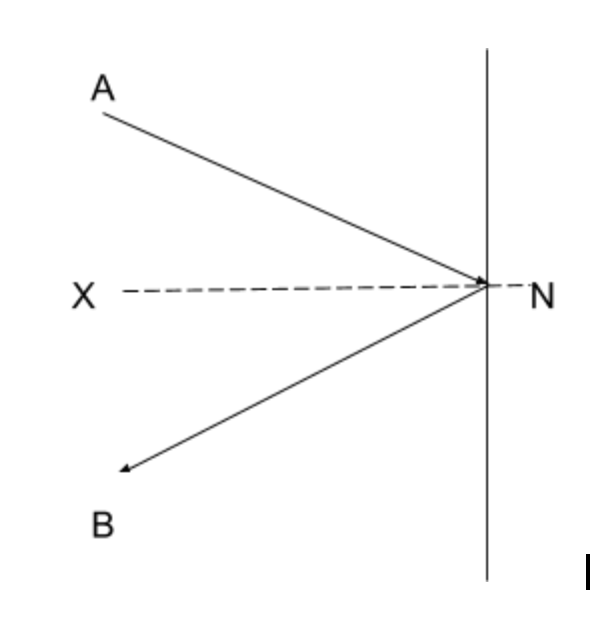D

#### Vectors in physics

9 viewed last edited 3 years agoManomay Shravage
1

An object of mass mkg with speed of vm/s strikes a wall at an angle theta with the normal and rebounds at the same speed and same angle. Calculate the resultant velocity in the direction perpendicular to the wall.Vivekanand Vellanki
2

Drawing a figure helps a lot in such situations. Let AN be the direction in which the object is travelling and hits the wall at point N. Let NB be the direction in the object travels after hitting the wall.

Laws of reflection tell us that \angle ANX\ =\angle XNB. In this both equal \theta.Before the object hit the ball, its magnitude in the direction XN (perpendicular to the wall) is: v\theta. The direction is from X to N.

After the object hit the ball, its magnitude perpendicular to the wall is: v\theta. However, the direction is now from N to X.

The resultant velocity perpendicular to the wall is v\theta m/s.Mahesh Godavarti
1

The magnitude of the final velocity perpendicular to the wall would be equal to v \cos \theta .

The change in velocity perpendicular to the wall would be equal to 2 v \cos \theta .